For the construction of the road dam, it is supposed to transport six trucks a total of 18 days. After three days, two more cars were sent to the construction site. How long will it take to carry the material?

Result

t =  14.25 d

Solution:

6*18 = 3*6+(t-3)*(6+2)

8t = 114

t = 574 = 14.25

Calculated by our simple equation calculator.

Leave us a comment of this math problem and its solution (i.e. if it is still somewhat unclear...):Be the first to comment!To solve this verbal math problem are needed these knowledge from mathematics:

Do you have a linear equation or system of equations and looking for its solution? Or do you have quadratic equation?

Next similar math problems:

1. WorkersTwo workers will construct the stage at the festival in 54 hours. Organizers need to shorten the assembly time by 42 hours. How many workers must the assembly company send?
2. Hectoliters of waterThe pool has a total of 126 hectoliters of water. The first pump draws 2.1 liters of water per second. A second pump pumps 3.5 liters of water per second. How long will it take both pumps to drain four-fifths of the water at the same time?
3. Working together on projectTwo employees working on the project. First make it alone for 20 days the second for 24 days. How many days will take to complete project if 5 days they work together then first will take two days holiday and after returning they completes a project toget
4. Lumberjacks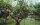Fifteen lumberjacks would take 12 days to destroy the broken trees. How many days would this work require if 5 workers added to it after 4 days?
5. The factoryThe factory z can complete an order in 12 days, factory p in 18 days. For how many days does an order completed when the first two days work only the factory z and then both factories?
6. Dwarfs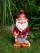Four dwarfs would prepare firewood for Snow White in580 minutes. After an hour and a half they recruit friends so that finished preparing the wood for 280 minutes. How many dwarfs they recruited?
7. Two workersThe first worker would himself completed the work in 8 hours, the other in 6 hours. After two hours of joint work left the first worker to a doctor and other completed work himself. How many hours worked the other worker himself?
8. Strawberries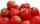Father collects strawberries himself in 4 hours, son in 14 hours. How long will it take them along when dad comes to help son collect strawberries after 3 hours?
9. Pumps A and BPump A fill the tank for 12 minutes, pump B for 24 minutes. How long will take to fill the tank if he is only three minutes works A and then both pumps A and B?
10. NovakNovak needed to dig up three of the same pit in the garden. The first pit dug father alone for 15 hours. His second dig son helped him and it did that in six hours. The third pit dug son himself. How long it took him?
11. Unknown numberIdentify unknown number which 1/5 is 40 greater than one tenth of that number.
12. Simple equationSolve for x: 3(x + 2) = x - 18
13. Sprayers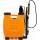The vineyard can be sprayed first sprayer in 5 hours and with the second in 8 hours. How long would it take if we use sprayers both at once?
14. EquationSolve the equation: 1/2-2/8 = 1/10; Write the result as a decimal number.
15. Six yearsIn six years Jan will be twice as old as he was six years ago. How old is he?
16. Collection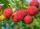John has collected half a bucket of raspberries in 45 minutes, Eva collects the whole bucket in an hour. How many minutes it would take to fill a bucket if the two children work together?
17. Welders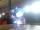Welder do the job for six hours, the second one for 8 hours. How long do the job third welder if all together can do job in 2 hours?